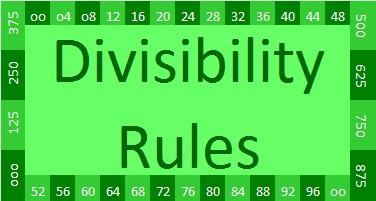# 140 and Gr-8 Divisibility Tricks

140 is a composite number. Factor pairs: 140 = 1 x 140, 2 x 70, 4 x 35, 5 x 28, 7 x 20, 10 x 14. Factors of 140: 1, 2, 4, 5, 7, 10, 14, 20, 28, 35, 70, 140. Prime factorization: 140 = 2 x 2 x 5 x 7, which can also be written 140 = 2² x 5 x 7.140 is never a clue in the FIND THE FACTORS puzzles.

——————————————————————-

In a previous post I discussed how to tell if a whole number can be evenly divided by 4, 25, or both just by looking at the last 2 digits of the number.

Mathematics is full of patterns so you might be wondering if there are divisibility tricks involving the last 3 digits of a number. Yes! There is!Again, because we use base 10, and 2 x 5 = 10, the following divisibility tricks work:

• 1000 (10 cubed) divides evenly into any number ending in 000.
• 125 (5 x 5 x 5) divides evenly into any whole number that ends with 000, 125, 250, 375, 500, 625, 750, 875. [I remember all 8 endings by thinking about U.S. coins. (000) no quarters equals 00 cents, (125) 1 quarter equals 25 cents, (250) 2 quarters equals 50 cents, (375) 3 quarters equals 75 cents. Adding 500 to each of those endings will give us the rest.]
• 8 (2 x 2 x 2) will divide evenly into whole numbers whose last 3 digits are divisible by 8.

Let’s explore that divisibility rule for eights a little more:

• There are 125 different three digit endings that are divisible by 8. I will not list them here, but here is a trick to the trick.

A whole number whose 3rd to the last digit is EVEN is divisible by 8 if the last 2 digits are (00, 08, 16, 24, 32, 40, 48, 56, 64, 72, 80, 88, 96)

• If you know the times tables up to 8 x 12, then you recognize ALL of those 2 digit numbers.
• If the 3rd to the last digit is odd and the last 2 digits are divisible by 4 but not by 8 (04, 12, 20, 28, 36, 44, 52, 60, 68, 76, 84, 92), then the whole number is divisible by 8. This last rule can be expressed more concisely using those same 8’s multiplication facts listed above:

A whole number whose 3rd to the last digit is ODD is divisible by 8 if its last two digits ± 4 = (00, 08, 16, 24, 32, 40, 48, 56, 64, 72, 80, 88, 96)

I like playing with these tricks, and they save me time especially when it comes to factoring larger whole numbers. I hope you will enjoy playing with them as well!